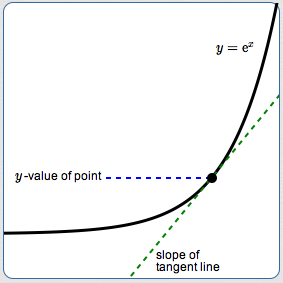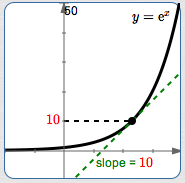﻿ A Special Property of the Natural Exponential Function

# A SPECIAL PROPERTY OF THE NATURAL EXPONENTIAL FUNCTION

by Dr. Carol JVF Burns (website creator)
Follow along with the highlighted text while you listen!
Thanks for your support!
• PRACTICE (online exercises and printable worksheets)

Have you been following my lessons in order?
If so, then you've heard me say (many times):

Exponential functions get big fast!

The special property of the natural exponential function discussed in this section will give you new appreciation for this fact!

## The $y$-value of a Point on the Graph of the Natural Exponential Function tells you the Slope of the Tangent Line

 Usually, calculus is needed to find slopes of tangent lines. (In particular, the derivative gives this information.) But...for the curve $\,y = {\text{e}}^x\,$ (which is the natural exponential function) finding slopes of tangent lines is easy! The slope of the tangent line at a point is just the $\,y\,$-value of the point!For the curve $\,y = {\text{e}}^x\,$: $y$-value of point $=$ slope of tangent line

The sketches below show just how simple things are for the natural exponential function!

If you know the $\,y\,$-value of a point, then you know the slope of the tangent line at that point.
If you know the slope of the tangent line at a point, then you know the $\,y\,$-value of the point.At this point, the $\,y\,$-values are changing $\,\color{red}{5}\,$ times faster than the $\,x\,$-values. At this point, the $\,y\,$-values are changing $\,\color{red}{10}\,$ times faster than the $\,x\,$-values. At this point, the $\,y\,$-values are changing $\,\color{red}{20}\,$ times faster than the $\,x\,$-values. At this point, the $\,y\,$-values are changing $\,\color{red}{30}\,$ times faster than the $\,x\,$-values. If $\,x\,$ changes by $\,0.1\,$,we expect $\,y\,$ to changeby about $\,5(0.1) = 0.5\,$. If $\,x\,$ changes by $\,0.1\,$,we expect $\,y\,$ to changeby about $\,10(0.1) = 1\,$. If $\,x\,$ changes by $\,0.1\,$,we expect $\,y\,$ to changeby about $\,20(0.1) = 2\,$. If $\,x\,$ changes by $\,0.1\,$,we expect $\,y\,$ to changeby about $\,30(0.1) = 3\,$.

When (say) $\,x = 10\,$, then $\,y = {\text{e}}^{10} \approx 22{,}026\,$.
At this point, the slope of the tangent line is about $\,22{,}026\,$.
At this point, the instantaneous rate of change is about $\,22{,}026\,$.
At this point, the outputs are changing about $\,22{,}026\,$ times as fast as the inputs!
Wow! And this is only when $\,x\,$ is $\,10\,$!
Exponential functions get big fast!

## For Those Who Know Some Calculus and Differential Equations

The derivative of $\,f(x) = {\text{e}}^x\,$ is $\,f'(x) = {\text{e}}^x\,$.
More generally, the derivative of $\,f(x) = K{\text{e}}^x\,$ is $\,f'(x) = K{\text{e}}^x\,$.
Notice that the derivative of the function is the same as the original function.
The $\,y\,$-values of points (given by the original function) and the slopes of tangent lines (given by the derivative) are the same.

We could ask:   What functions are the same as their derivatives?
Equivalently, we could ask:   What are the solutions of the differential equation $\,y = y\,'\,$?
Head up to WolframAlpha and type in:   solve y = y'
The family of solutions is $\,y = K{\text{e}}^x\,$ (one for each real number $\,K\,$).

Scalar multiples of the natural exponential function
are the ONLY functions
where finding slopes of tangent lines
is as easy as
reading off the $\,y\,$-values of points!

Master the ideas from this section
by practicing the exercise at the bottom of this page.

When you're done practicing, move on to:
simple versus compound interest
On this exercise, you will not key in your answer.
However, you can check to see if your answer is correct.
PROBLEM TYPES:
 1 2 3 4 5
AVAILABLE MASTERED IN PROGRESS
 (MAX is 5; there are 5 different problem types.)